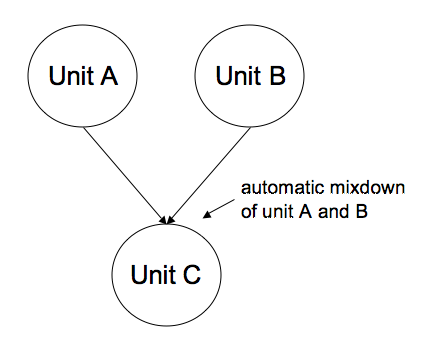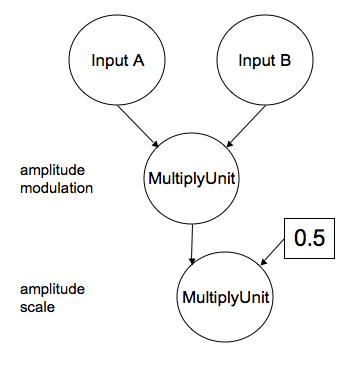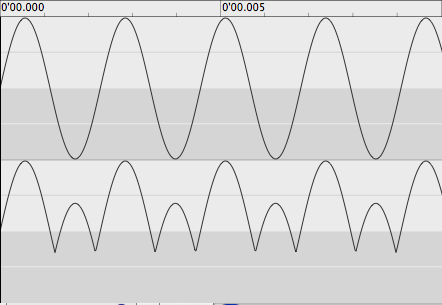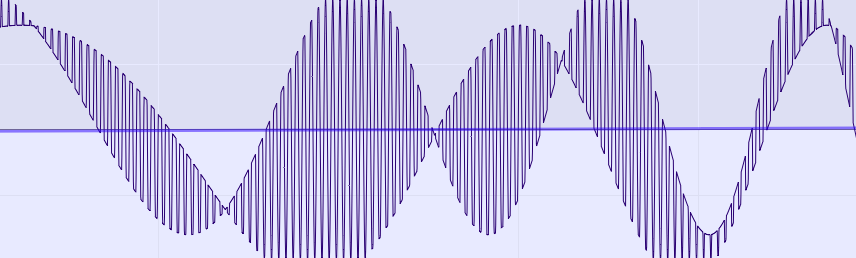Interactive Swarm Space

Binary Operator Units

You guessed it right, AddUnit lets you add two signals together or add a specific value to one input signal (amplitude offset). The first case is actually rather rarely used when creating patches because all units have the basic capability of mixing input signals automatically when received on the same port.multiple inputs on one port are added (mixed) together.

So AddUnit is most useful when offsetting a signal, e.g. to correct an existing DC offset.

In this example we scale and offset an audio file, so that it will play in the amplitude range from 0.0 to 1.0.

```InputFile* input = new InputFile("<directory_to_the_file>/test_sound.aif");

input->set("amplitude", 0.5); // half the amplitude, right now the file will have
// the range [-0.5, 0.5]
add->set("operand", 0.5); //+ 0.5 offset

```

MultiplyUnit

Similar to AddUnit, MultiplyUnit has two usages. You can use it to multiply two signals with each other (amplitude modulation). Or you can use it to scale an input signal by a defined factor. On a side note, these two cases are internally the same. In both cases, the operand is a buffer - in the first cases the buffer is constantly filled by a second audio input and in the second case the whole buffer holds the same value.

Here is a code example for both cases.

```InputFile* input1 = new InputFile("<directory_to_the_file>/test_sound.aif");
InputFile* input2 = new InputFile("<directory_to_the_file>/another_test_sound.aif");
MultiplyUnit* ampModulator = new MultiplyUnit();
MultiplyUnit* ampScale = new MultiplyUnit();

ampScale->set("operand", 0.5);

input1->connect(ampModulator);
input2->connect(ampModulator, "operand"); // not the default input, hence the "operand"
// string, defining the target input port

ampModulator->connect(ampScale);

ampScale->connect(output);
```schematic of the two basic usages for the MultiplyUnit

Hint

It is maybe worth mentioning again that every unit has a built-in output gain. So for regular amplitude scaling needs it's easiest to simply call:

`someUnit->set("outputGain", 0.5); //scales the output by 0.5 `

AbsUnit

AbsUnit is a ProcessUnit that will output the absolute values of the data. As an addition, you can define the offset threshold bellow which all values are mirrored upwards.

Hint

To invert the output of AbsUnit, simply it's "outputGain" SwitchPort to "-1.0" (a method that works in fact with all units).

```WaveTableOscil* osc = new WaveTableOscil("sinewave");AbsUnit* abs = new AbsUnit();
abs->set("offset", -0.3);
osc->connect(abs);
abs->connect(outputUnit);
```Above: input sinewave signal; Below: output of AbsUnit with an offset of -0.3

LimiterUnit

Occasionally you might want to truncate your signal. ISOSynth offers a very versatile tool for this. The LimiterUnit lets you define two threshold values, everything outside these two values is truncated (set to the effective value of the threshold) . As with all control input to ISOSynth's units, these thresholds can be controlled in realtime. The following example demonstrates this. Three wavetables are used, one as carrier oscillator and two to control the thresholds. The order of these two thresholds is of no importance, ISOSynth detects itself, which one is the upper threshold, and which one the lower one.
```WaveTableOscil* input = new WaveTableOscil("sinewave");
WaveTableOscil* threshMod1 = new WaveTableOscil("sinewave");
WaveTableOscil* threshMod2 = new WaveTableOscil("sinewave");
LimiterUnit* lim = new LimiterUnit();

input->set("frequency", 500.0);

threshMod1->set("frequency", 2.22222);
threshMod2->set("frequency", 1.1);
threshMod1->set("offset", 0.2);
threshMod2->set("offset", -0.2);

input->connect(lim);
thresh1->connect(lim, "thresh1");
thresh2->connect(lim, "thresh2");

lim->connect(m_output);
```modulated limits using two sine wavetables to control a LimiterUnit

In case you just need a fixed value as threshold, you can set it by calling:

```lim->set("thresh1", 0.8);
lim->set("thresh2", -0.8);```

DeltaUnit

DeltaUnit calculates discrete derivatives of a signal in realtime. Or in the language of mere mortals: the change of a signal (first order) or the change of the change (2nd order) and so on:

Order 0: deltat0 = signalt0
Order 1: deltat0 = signalt0 - signalt-1
Order 2: deltat0 = (signalt0 - signalt-1) - (signalt-1 - signalt-2)
...

While using DeltaUnit, you will notice that because of the nature of this unit, it will attenuate low-frequent steady state signals while amplifying noisy or high-frequent signals, which can serve as a sort of transient filter.

The higher the order the more the DeltaUnits needs to look back in its buffer (or the previous buffer internally). The highest derivative order has the same value as the units internal frame rate per process buffer (512 by default).

```Noise* inputSignal = new Noise();
DeltaUnit* delta = new DeltaUnit();
delta->set("order", 2.0); //2nd derivative
inputSignal->connect(delta);
delta->connect(outputUnit);```